output.to from Sideway
Draft for Information Only

# Content

`Coulomb's law Electrostatic Force Electric Field Source and Reference`

# Coulomb's law

Coulomb's law or Coulomb's inverse-square law is a low of physics used to quantify the amount of force between two stationary elctrically charged particles.

## Electrostatic Force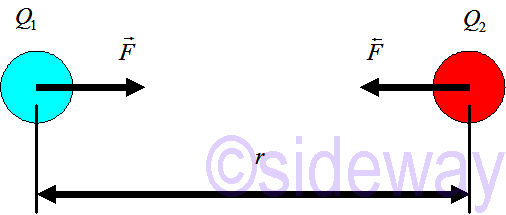Electrostatic force or Coulomb force is defined as the electric force between two charged bodies at rest. The quantity of electrostatic force between stationary charges is always described by Coulomb's law.

`𝐹=14𝜋𝜀𝑒𝑄1𝑄2𝑟2𝑟=𝑘𝑒𝑄1𝑄2𝑟2𝑟`

The direction of force is attractive if charges have opposite sign as above and is repulsive if charges have same sign as below. The electrostatic force always acts along a line connecting tht charges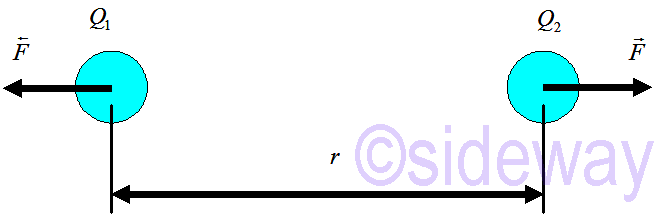The magnitude of electrostatic force is proportional to the magnitude of each charge and inversely proportional to the distance squared

`𝐹=𝐹=14𝜋𝜀𝑒|𝑄1𝑄2|𝑟2=𝑘𝑒|𝑄1𝑄2|𝑟2`

The unit of electric charge, 𝑄 in SI unit is Coulomb, 𝐶. The unit of electrostatic force, 𝐹 is 𝑁. The unit of distance, 𝑟 is 𝑚. Therefore the unit of permittivity, 𝜀 is 𝐶2/𝑁𝑚2.

The vacuum permittivity, 𝜀0 is equal to 8.85×10-12𝐶2/𝑁𝑚2=8.85×10-12𝐹𝑚-1. The Coulomb's constant, 𝑘𝑒 for vacuum, 1/4𝜋𝜀0 is 9×109𝑁𝑚2/𝐶2

The electric charge of an electron, 𝑒 is 1.602×10-19𝐶. The electric charge quantity of an electron, 𝑄 is −𝑒, while the electric charge quantity of a proton, 𝑄 is +𝑒.

## Electric Field

From Coulomb's law, a particle with electric charge, 𝑄0 at position, 𝑥0 exerts a force on a particle with electric charge, 𝑄1 at position, 𝑥1 can be expressed as `𝐹=14𝜋𝜀0𝑄0𝑄1(𝑥1−𝑥0)2𝑟` The effect of the source charged particles 𝑄0 at position 𝑥0 on charged particles, 𝑄1 at position 𝑥1can be expressed as `𝐹0,1𝑄1=14𝜋𝜀0𝑄0(𝑥1−𝑥0)2𝑟0,1` Since charged particles exerts electrostatic forces in all direction at all times, whether or not there is another charged particles around, the effect of the source charged particles at position, 𝑥 on charged particles, 𝑄 throughout space at all time can be expressed as `𝐸𝑥=𝐹𝑥𝑄=14𝜋𝜀0𝑄0(𝑥−𝑥0)2𝑟𝑥` This is the electric field which is equal to Electrostatic force per unit charge that a point charge 𝑞 of the same sign would exprerience at position 𝑥 due to the charged particles, 𝑄0 of positive charge 𝑞0 at position 𝑥0. The unit of electric field is Newton/Coulomb.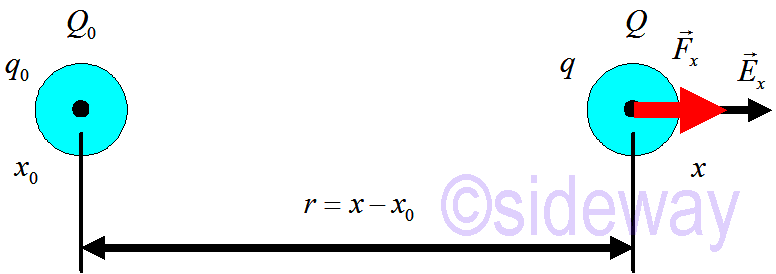In other words, the electrostatic force that acts on any charge can be expressed as `𝐹=𝑞𝐸=14𝜋𝜀0𝑞0𝑟2𝑟` The direction of electric field due to a charged particle is only affected by the sign of charges. A positive charged particle generates a pointing outward electric field, while a negative charged particle generates a pointing inward electric field.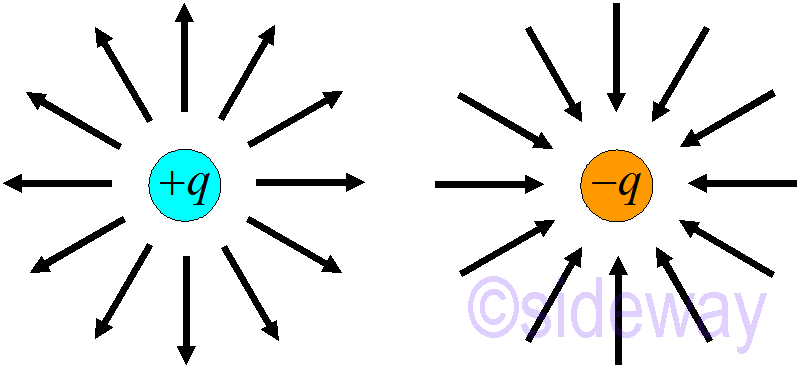The direction of electrostatic force acting on an charaged observed particle due to the electic field generated by a charged source particle depends on the signes of the two charged particles. However, a positively charged observed particle alway experences an electrostatic force which is along with the same direction of electric field, while a negatively charged observed particle particle alway experences an electrostatic force which is against the direction of electric field, i.e. in the opposite direction of electric field.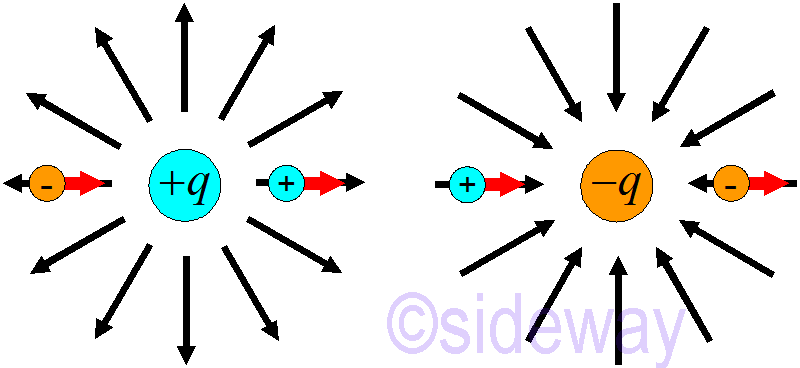## Source and Reference

ID: 191003002 Last Updated: 10/30/2019 Revision: 0Home 5

Management

HBR 3

Information

Recreation

Culture

Chinese 1097

English 339

Computer

Hardware 226

Software

Application 213

Latex 52

Manim 205

KB 1

Numeric 19

Programming

Web 289

Unicode 504

HTML 66

CSS 65

SVG 46

ASP.NET 270

OS 429

Python 72

Knowledge

Mathematics

Algebra 84

Geometry 34

Calculus 67

Engineering

Mechanical

Rigid Bodies

Statics 92

Dynamics 37

Control

Natural Sciences

Electric 27